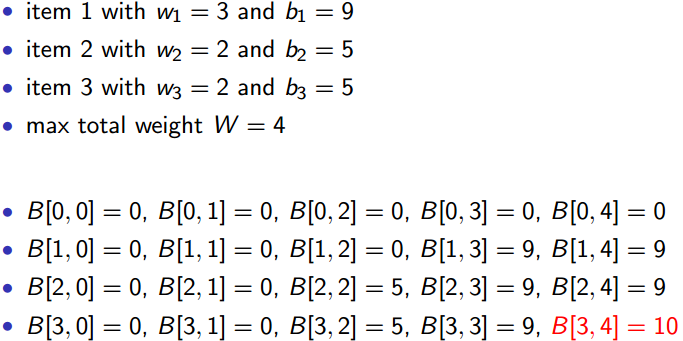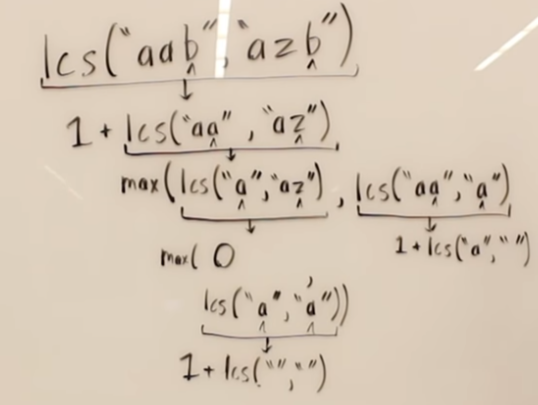# Dynamic Programming Part2

Updated:

⚠️ This post was created when I was in high school and is no longer maintained.

## 1. Fibonacci

Bottom-up solution:

algorithm FibonacciDP(n):
{- Not a recursive solution, so don't require an exit !
if n == 1 or n == 2 then
return 1
-}
let fib[1 ~ n] be a new array;
fib, fib = 1, 1
For i := 3 to n do
fib[i] = fib[i-1] + fib[i-2]
return fib[n]


O(n)

## 2. Max-subarray

### 2.1 Brute-force

In this naive solution, we try every possible combination of starting and ending indices, which will result in order of n^2^ time complexity.

algorithm MaxSubarrayNaive(array, len):
max := -inf
For left := 0 to len do
sum := 0
For right := left to len do
sum += array[right]
if sum > max then
max = sum
return max

• Time complexity: O(n^2^) (arithmetic progression)

### 2.2 DP method

Algorithm MaxSubArray(A, n):
Let B be a new array of size n;
B := max(0, A)
maxSoFar := 0   --> base case

For i:= 2 to n do
B[i] := max(0, B[i-1]+A[i])
maxSoFar := max(B[i], maxSoFar)

return maxSoFar


For my understanding, the essence of dynamic programming is to assume that the result of the previous step is always optimal. Consequently, before we start solving problems using DP, we need to find out a base case.

In this problem, it can be that the first element we choose is an optimal one (positive value or 0).

Then, in each step, we have to make 2 decisions. First off, whether or not we should keep the value of the current position: B[i] := max(0, B[i-1]+A[i])

If the summation of the current value A[i] and the previous optimal result is negative, then the A[i] definitely has no contribution to the optimal solution, so we drop it by setting B[i] to 0, which means we cut off the previous subarray from here and start a new subarray accumulation from the next value.

Secondly, we should see if the current optimal choice will contribute to the overall result: maxSoFar := max(B[i], maxSoFar)

As a final note, I think this algorithm won’t work if all the elements in the input array are negative because the return value will always be 0. To make it work for this circumstance, a few changes are needed.

## 3. The 0-1 knapsack problem

Unlike the fractional knapsack problem which can be solved by the greedy strategy, the 0-1 problem cannot be solved by such an algorithm, although both knapsack problems exhibit the optimal-substructure property.

### 3.1 Pseudocode

struct Item {
int weight;
double benifit;
} item;

items := Array[Item](n)

Algorithm 01Knapsack(items, weightLimit):
New dpTable[0 ~ n][0 ~ weightLimit]
n := items.length

For i := 0 to n do
dpTable[i] := 0 --> initial DP base case

For k := 0 to n do
dpTable[k] := 0 --> initial base case for each item

For wl := 1 to weightLimit do:
if items[k].weight <= wl then
dpTable[k][wl] := Max(dpTable[k-1][wl], dpTable[k][wl - items[k].weight] + items[k].benifit)
else
dpTable[k][wl] := dpTable[k-1][wl]

return dpTable[n][weightLimit]


### 3.2 Intuition

• As the code implied above, what we are doing is just building up a DP table in a bottom-up manner.

• Each cell dpTable[k][w] holds the maximum benefit we could possibly get when there are 0 to k numbers of elements available and the weight limit of the knapsack is w.

• In the instruction: Max(dpTable[k-1][wl], dpTable[k][wl - items[k].weight] + items[k].benifit), we make a decision that whether should we pick the kth item, item[k],

For instance,( here, B[ , ] <=> dpTable[][] )

### 3.3 Time complexity

The time complexity of 01knapsack problem lies in the size of the dpTable which is n * weightLimit. Consequently, if the weightLimit is something like 2^n^ then T(n) will not be nice… In a nutshell, this is a pseudo-polynomial algorithm

## 4. Longest Common Subsequence

### 4.1 Intuition of chopping two strings:### 4.2 Intuition of the DP table:

/*
This is what the DP table looks like filled out for the 2 strings
"AGGTAB" and "GXTXAYB".

""  A  G  G  T  A  B
""  0  0  0  0  0  0  0
G   0  0  1  1  1  1  1
X   0  0  1  1  1  1  1
T   0  0  1  1  2  2  2
X   0  0  1  1  2  2  2
A   0  1  1  1  2  3  3
Y   0  1  1  1  2  3  3
B   0  1  1  1  2  3  4

Each cell is a subproblem call on the
appropriate substring snippets.

For example, table[G][G] represents
the function call LCS("AG", "G")
*/


Tags: